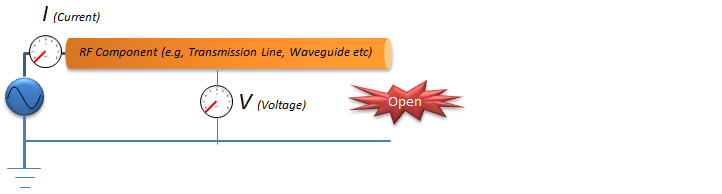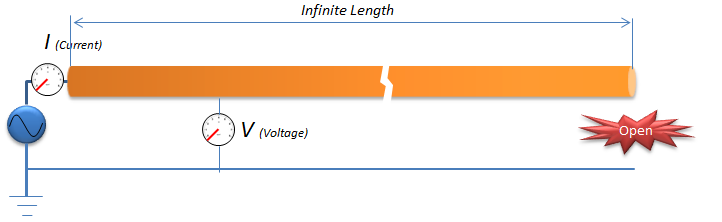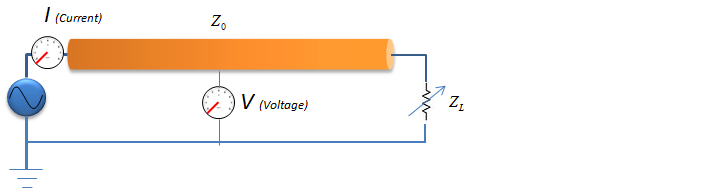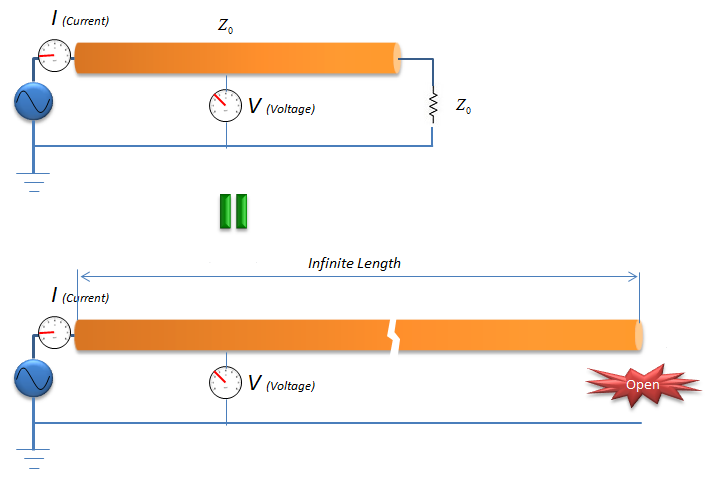RF - Characteristic Impedance                                         Home : www.sharetechnote.com Characteris Impendance is one of the terms we see the most often and use ourselves, but very vague and hard to explain. Following is some of the definition of Characteristic Impendance from several different sources. (If you check 10 different sources, you would see 10 different variations of description). Characteristic Impendance is the impedance of a circuit that, when connected to the output terminals of a uniform transmission line of arbitrary length, causes the line to appear infinitely long The characteristic impedance or surge impedance (usually written Z0) of a uniform transmission line is the ratio of the amplitudes of voltage and current of a single wave propagating along the line; that is, a wave travelling in one direction in the absence of reflections in the other direction Characteristic Impedance is the instantaneous impedance a signal sees as it moves down the line.   Does this make sense to you ? It would make sense if you already know what Characteristic Impendance is.. but it would not make much sense if this is new to you. It was like that to me when I first looked at it. Probably there wouldn't be any way to get you the clear understanding of the concept with a couple of lines of writing. Just try to read through many different versions of explanation and you would get more and more familiar with the concept and then you would gradually catch the real meaning of it even though it would still be hard for you to explain it to somebody else. My explanation also can be only one version of many different explanation you would get from different sources, I don't expect just reading my explanation once or twice would give you complete understanding of the concept of Characteristic Impedence.   Let's suppose that you have a circuit as shown below. What would happen in the current meter (Ampere meter) and voltage meter when you apply the input source ? If you think of what you learned in high school physics, the answer would be simple. You would say '0' in both Ampere meter and Voltimeter since the circuit is open (broken in one end). However if you think a little bit deep and think of the situation in very short time scale like nano or pico second interval. If you break down the time in pico second scale, you may be able to say 'I would see some current and voltage during a couple of pico second right after I apply the source because the current would get out of the source and flow through the RF component until it reaches the end of the circuit. The current would stop flowing when it reaches to the end of the circuit.Applying the concept described above, you would see the current and voltage for loger and longer as you use longer and longer RF Component because it takes longer time for the current from the source to reach the end of the circuit. If we assume that we can extend the length of the RF component to inifinite length, the current would flow forever even though the circuit is open at the end because it would take forever for the current from the source to reach the end of the circuit. In this case, the current would flow in only one direction from the source to the end of the RF component because there would be no reflection from the end of the component. Assuming that the reflection happens only at the end of the component, there would be no chance for the signal get reflected because it would take infinate time (meaning never happens) to reach the end of the component.   If you measure the current and voltage in this kind of ideal condition, you can calculate the impedance as follows. Z = V/I The Z (impendence) measured in this kind of ideal condition is called 'Characteristic Impedence' because the measured value is determined by 'physical/electrical characteristics of the RF component (e.g, material, physical dimension, shape etc).Of course, you cannot build this kind of ideal circuit because you cannot make any RF component with infinite length.   So let's think of more practical approach. Let's suppose you have a circuit as shown below. In this circuit, the circuit is not open, now it is a closed circuit and it is closed by a load labeled as Z_L (load impedance).Assume that you just put any arbitrary value for the load impedance and you will see a certain value at Ampere meter and Voltimeter. However, in most case the value you read in the meter would not be same as the one you would see in the ideal case that I described above because some portions of the signal (source power) would get reflected at the end of the RF component. With a lot of trials and a lot of luck (?), you may find a specific Z_L value at which you see the same Ampere meter value and Votimeter value as in the ideal case described above, the specific Z_L value at this condition become same as the characteristic impedance of the component. It means that the Z_L (Load Impedance) create the same effect of lengthening the RF component to the ininite length. (This is the meaning of the first definition at the beginning of this page)Now go back to the beginning of this page and read the sample definition of Characteristic Impedance and see if it make sense to you. If it does not make clear sense to you yet, read some other materials as listed below and try whatever you can search from google or other text book.     Further Reading